# GSEB Solutions Class 6 Maths Chapter 13 Symmetry InText Questions

Gujarat Board GSEB Textbook Solutions Class 6 Maths Chapter 13 Symmetry InText Questions and Answers.

## Gujarat Board Textbook Solutions Class 6 Maths Chapter 13 Symmetry InText Questions

Try These (Page 262)

Question 1.
You have two set-squares in your mathematical instruments box. Are they symmetrical?
Solution:
The two set-squares are given as: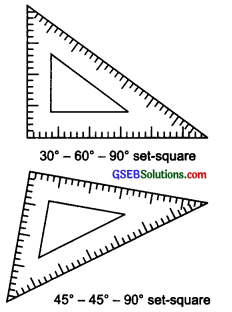The 30° – 60° – 90° set square is not symmetrical.
The 45° – 45° – 90° set square is symmetrical.Try These (Page 264)

Question 1.
Form as many shapes as you can by combining two or more set-squares. Draw them on squared paper and note their lines of symmetry.
Solution:
(i) By combining two identical 30°-60°-90° set squares, we get the following symmetric figures:

(a) l1 and l2 are the lines of symmetry.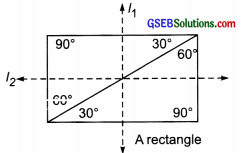(b) Here, there is only one line of symmetry which is shown by ‘m’.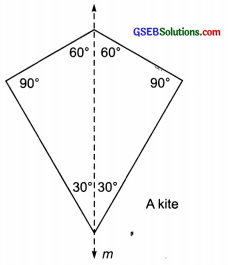(c) Here, the dotted line ‘q’ is the only line of symmetry.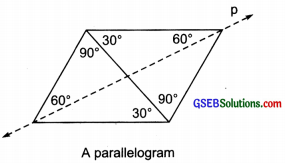(d) Here, the dotted line ‘q’ is the only line of symmetry.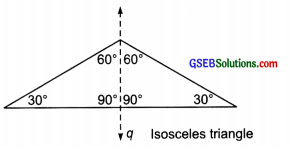(ii) By combining two identical 45° – 45° – 90° set- squares, we get the following symmetric figures:

(a) Here, l1, l2, l3, l4 are lines of symmetry.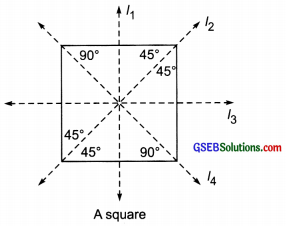(b) In the figure formed, there is only one line of symmetry which is shown by ‘r’.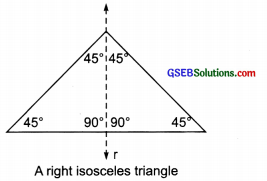(iii) By combining three 45° – 45° – 90° identical set-squares, we can have an isosceles trapezium as shown here: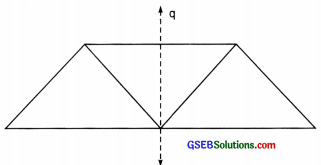Here, the line of symmetry is shown by the dotted line ‘q’.Try These (Page 270)

Question 1.
If you are 100 cm in front of a mirror, where does your image appear to be? If you move towards the mirror, how does your image move?
Note: In the mirror reflection, there exists a lateral difference between the object and the image. The lateral difference means the left part of the object becomes the right part of the image and vice versa.
Solution:
In a mirror, an image is as far behind the mirror as the object is in front of it. Your image will appear at 100 cm behind the mirror. If you move towards the mirror the image will also move closer.